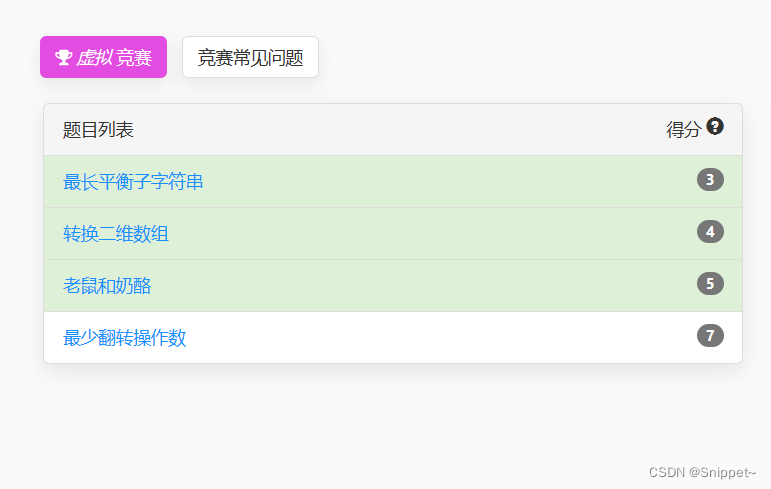# 蓝桥杯30天真题冲刺|题解报告|第三十天

一、最长平衡子字符串### 一、最长平衡子字符串

#### 题目内容：

1 <= s.length <= 50

'0' <= s[i] <= '1'

#### 解题思路：

1.前面没有1，0的数量++；

2.前面有1，重新计数；

1.前面没有0，直接countinue跳转到下一个字符进行计算

2.前面有0，前面有0的话，需要计算0和1的个数，ans = Math.min(cnt0,cnt1)*2；

#### 代码：

package 蓝桥杯30天真题冲刺.Day30.力扣第339场周赛;

import java.io.*;

/**
* @author snippet
* @data 2023-04-02
* 力扣第399场周赛-最长平衡子字符串
*/
public class T1_最长平衡子字符串 {
static PrintWriter pw = new PrintWriter(new BufferedWriter(new OutputStreamWriter(System.out)));

public static void main(String[] args) throws IOException {

pw.flush();
br.close();
}

public int findTheLongestBalancedSubstring(String s) {
if (s == "") return 1;
int ans = 0;
int cnt0 = 0;
int cnt1 = 0;
for (int i = 0; i < s.length(); i++) {
if (s.charAt(i) == '0') {
if (cnt1 != 0) {
cnt1 = 0;
cnt0 = 1;
} else {
cnt0++;
}
} else {
if (cnt0 == 0) continue;
cnt1++;
ans = Math.max(ans, Math.min(cnt0, cnt1)*2);
}
}
return ans;
}
}


### 二、转换二维数组

#### 题目内容：

1.二维数组应该 只 包含数组 nums 中的元素。

2.二维数组中的每一行都包含 不同 的整数。

3.二维数组的行数应尽可能 少 。

- 1,3,4,2
- 1,3
- 1
nums 中的所有元素都有用到，并且每一行都由不同的整数组成，所以这是一个符合题目要求的答案。

1 <= nums.length <= 200

1 <= nums[i] <= nums.length

#### 代码：

package 蓝桥杯30天真题冲刺.Day30.力扣第339场周赛;

import java.io.*;
import java.util.*;

/**
* @author snippet
* @data 2023-04-02
* 力扣第399场周赛-转换二维数组
*/
public class T2_转换二维数组 {
static PrintWriter pw = new PrintWriter(new BufferedWriter(new OutputStreamWriter(System.out)));

public static void main(String[] args) throws IOException {

pw.flush();
br.close();
}

public List<List<Integer>> findMatrix(int[] nums) {
Map<Integer, Integer> map = new HashMap<>();
List<List<Integer>> list = new ArrayList<>();
int len = nums.length;
int max = 0;
for (int i = 0; i < len; i++) {
}
for (int i = 0; i < len; i++) {
max = Math.max(nums[i], max);
map.put(nums[i], map.getOrDefault(nums[i], 0)+1);
}
for (List<Integer> temp : list) {
for (int i = 1; i <= max; i++) {
if (map.containsKey(i) && map.get(i) != 0) {
map.put(i, map.get(i)-1);
}
}
}
List<List<Integer>> ans = new ArrayList<>();
for (List<Integer> temp : list) {
if (temp.size() != 0) {
}
}
return ans;
}
}


### 三、老鼠和奶酪

#### 题目内容：

1.如果第一只老鼠吃掉，则得分为 reward1[i] 。

2.如果第二只老鼠吃掉，则得分为 reward2[i] 。

15 是最高得分。

2 是最高得分。

1 <= n == reward1.length == reward2.length <= 10^5

1 <= reward1[i], reward2[i] <= 1000

0 <= k <= n

#### 代码：

package 蓝桥杯30天真题冲刺.Day30.力扣第339场周赛;

import java.io.*;
import java.util.*;

/**
* @author snippet
* @data 2023-04-02
* 力扣第399场周赛-老鼠和奶酪
*/
// 贪心
public class T3_老鼠和奶酪 {
static PrintWriter pw = new PrintWriter(new BufferedWriter(new OutputStreamWriter(System.out)));

public static void main(String[] args) throws IOException {
int[] a = {1,4,4,6,4};
int[] b = {6,5,3,6,1};
System.out.println(miceAndCheese(a,b,1));
pw.flush();
br.close();
}

static public int miceAndCheese(int[] reward1, int[] reward2, int k) {
int sum = 0;
Integer[] arr = new Integer[reward1.length];
for (int i = 0; i < reward1.length; i++) {
arr[i] = reward1[i] - reward2[i];
sum += reward1[i];
}
Arrays.sort(arr);
for (int i = 0; i <reward1.length- k; i++) {
sum -= arr[i];
}
return sum;
}
}


09-251190
09-191395
09-2785
09-22527
09-212565
09-214175
09-262463
11-12
09-25249
09-191388
06-08
05-15
09-22512
09-27141
09-20344
09-29551
09-21381

### “相关推荐”对你有帮助么？

•非常没帮助
•没帮助
•一般
•有帮助
•非常有帮助被折叠的  条评论 为什么被折叠?到【灌水乐园】发言Snippet~

¥1 ¥2 ¥4 ¥6 ¥10 ¥20获取中扫码支付点击重新获取扫码支付1.余额是钱包充值的虚拟货币，按照1:1的比例进行支付金额的抵扣。
2.余额无法直接购买下载，可以购买VIP、付费专栏及课程。余额充值